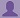SHARE
SPREAD
HELP

### The Tradition of Sharing

#### Help your friends and juniors by posting answers to the questions that you know. Also post questions that are not available.

To start with, Sr2Jr’s first step is to reduce the expenses related to education. To achieve this goal Sr2Jr organized the textbook’s question and answers. Sr2Jr is community based and need your support to fill the question and answers. The question and answers posted will be available free of cost to all.# Computer Networking : A Top-down Approach

Authors:
James F. Kurose, Keith W. Ross
Exercise:
Problems
Chapter:
Computer Networks And The Internet
Edition:
6
ISBN:
9780132856201
Question:
6
 Previous Next

###Question

This elementary problem begins to explore propagation delay and transmission delay, two central concepts in data networking. Consider two hosts, A and B, connected by a single link of rate R bps. Suppose that the two hosts are separated by m meters, and suppose the propagation speed along the link is s meters/sec. Host A is to send a packet of size L bits to Host B.

a. Express the propagation delay, dprop, in terms of m and s.

b. Determine the transmission time of the packet, dtrans, in terms of L

and R.

c. Ignoring processing and queuing delays, obtain an expression for the end-to-end delay.

d. Suppose Host A begins to transmit the packet at time t = 0. At time t = dtrans,where is the last bit of the packet?

e. Suppose dprop is greater than dtrans. At time t = dtrans, where is the first bit of the packet?

f. Suppose dprop is less than dtrans. At time t = dtrans, where is the first bit of the packet?

g. Suppose s = 2.5 · 108, L = 120 bits, and R = 56 kbps. Find the distance m so that dprop equals dtrans.

###Answer

a) The propagation delay, dprop=m/s sec

b) The transmission time of the packet, dtrans=L/R sec

c)  The end-to-end delay=(L/R+m/s) sec

d) Suppose Host A begins to transmit the packet at time = 0. At time dtrans.

Then, the last bi of the packet dtrans

e) Suppose dprop is greater than dtrans. At time dtrans

Thus, the first bit of the packet is dpropdtrans.

f)Suppose dprop is less than dtrans. At time dtrans,

Thus, the first bit of the packet is dpropdtrans.

###vamshi banoth

answers for:

d)The bit is just leaving Host A

e)The first bit is in the link and has not reached Host B

f)The first bit has reached Host B

Post the discussion to improve the above solution.